Ex 7.3

Chapter 7 Class 8 Comparing Quantities
Serial order wise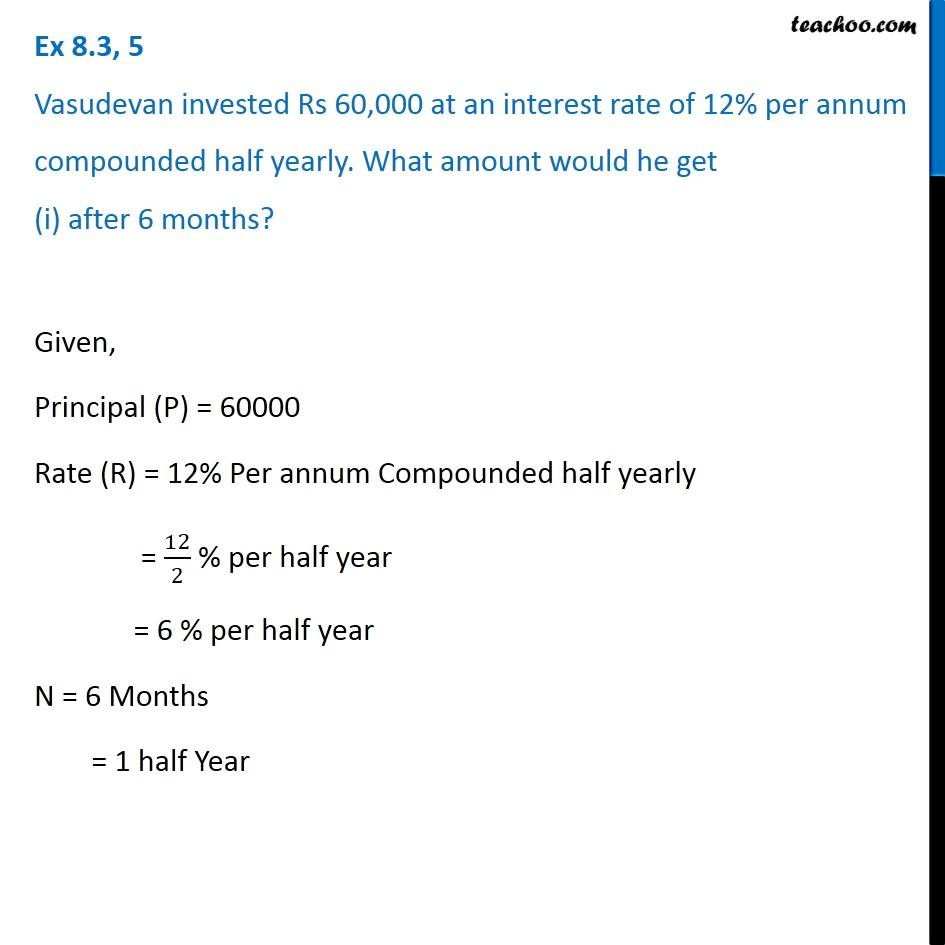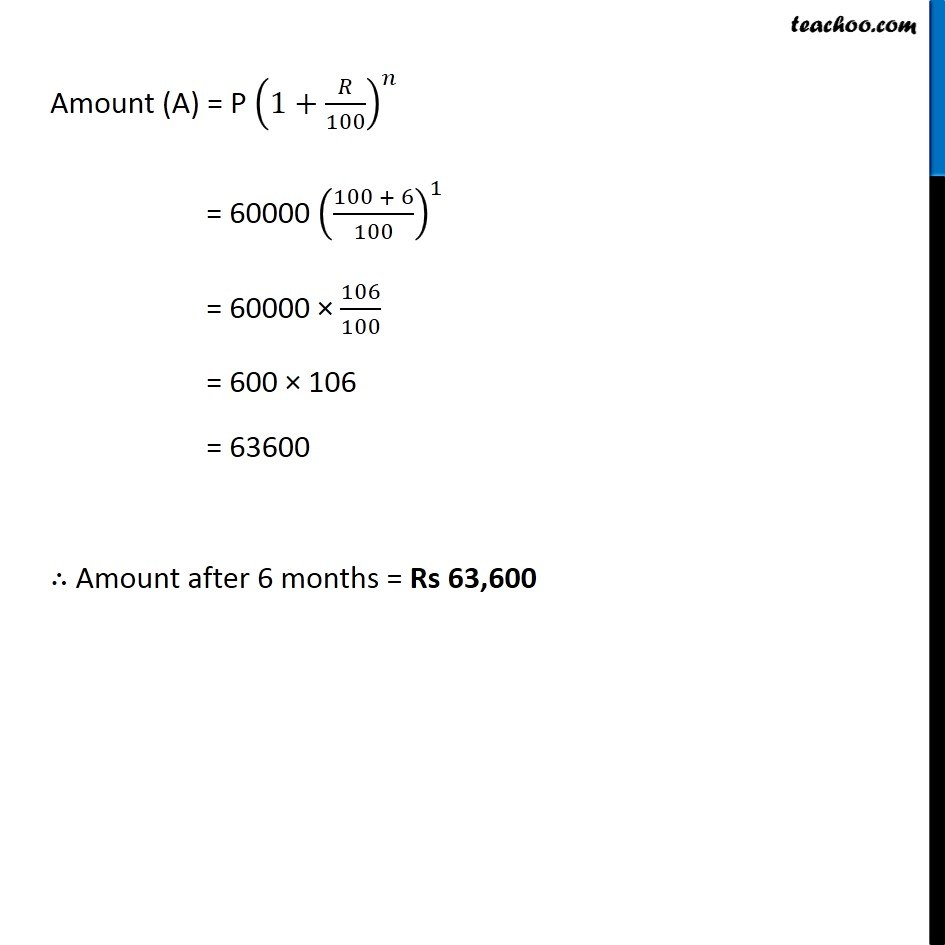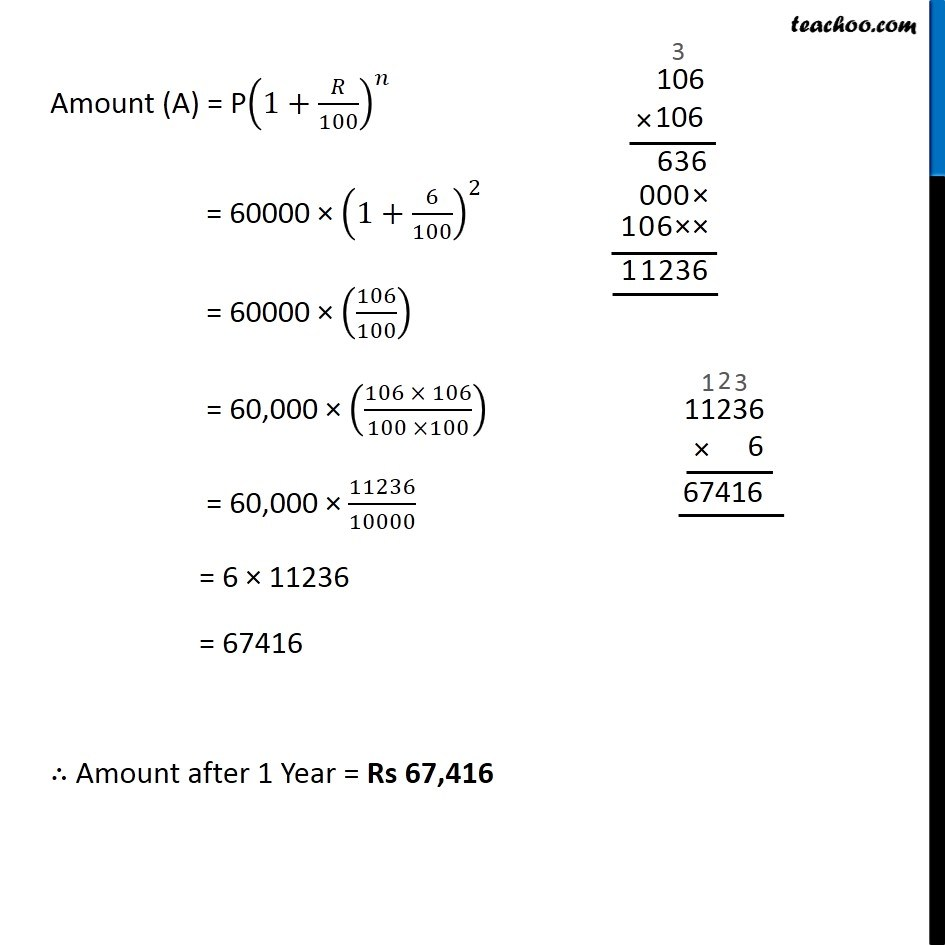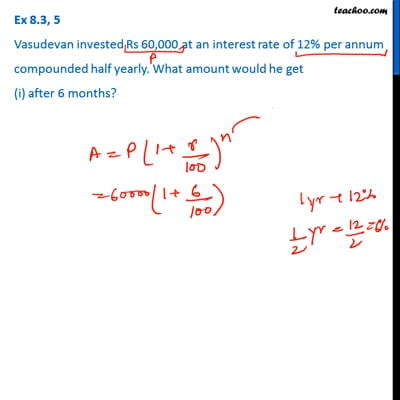This video is only available for Teachoo black users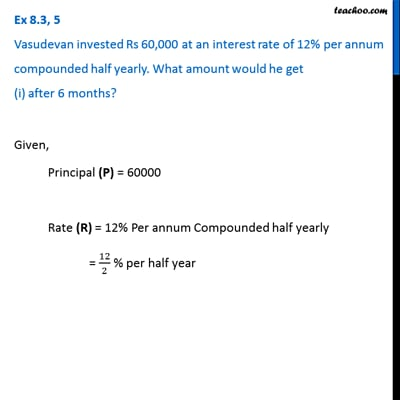This video is only available for Teachoo black users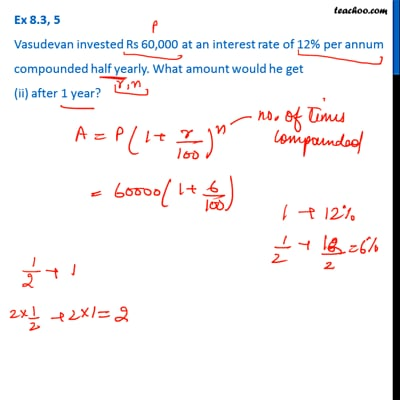This video is only available for Teachoo black users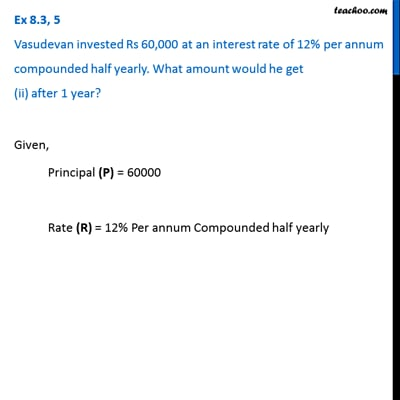This video is only available for Teachoo black users

Learn in your speed, with individual attention - Teachoo Maths 1-on-1 Class

### Transcript

Question 5 Vasudevan invested Rs 60,000 at an interest rate of 12% per annum compounded half yearly. What amount would he get (i) after 6 months? Given, Principal (P) = 60000 Rate (R) = 12% Per annum Compounded half yearly = 12/2 % per half year = 6 % per half year N = 6 Months = 1 half Year Amount (A) = P (1+𝑅/100)^𝑛 = 60000 ((100 + 6)/100)^1 = 60000 × 106/100 = 600 × 106 = 63600 ∴ Amount after 6 months = Rs 63,600 Question 5 Vasudevan invested Rs 60,000 at an interest rate of 12% per annum compounded half yearly. What amount would he get (ii) after 1 year? Given, Principal (P) = 60000 Rate (R) = 12% Per annum Compounded half yearly = 12/2 % per half year = 6 % per half year n = 1 Year = 2 Half years Amount (A) = P(1+𝑅/100)^𝑛 = 60000 × (1+6/100)^2 = 60000 × (106/100) = 60,000 × ((106 × 106)/(100 ×100)) = 60,000 × 11236/10000 = 6 × 11236 = 67416 ∴ Amount after 1 Year = Rs 67,416